# Analog Circuit Based on the Shock Pulse Method and Its Application in Fault Diagnosis of Bearing

Analog Circuit Based on the Shock Pulse Method and Its Application in Fault Diagnosis of Bearing

Yanzhong Li Zheng Liu Zengqiang Ma

School of Electrical and Electronics Engineering, Shijiazhuang Tiedao University, Shijiazhuang 050043, China

Corresponding Author Email:
06116272@stdu.edu.cn
Page:
35-38
|
DOI:
https://doi.org/10.18280/mmep.030106
N/A
|
Accepted:
N/A
|
Published:
31 March 2016
| Citation

OPEN ACCESS

Abstract:

There are many factors which can effect the detection of bearing defect. Noise is a very important factor. Too much noise will lead negative effect to the detection of bearing defect, which will lead to a result that the fault can’t be detected. In the study of state judgement of rolling bearing, the shock pulse method is the most commonly and widely used. However, the shock pulse signal generated by the rolling bearing is different from that of the ordinary machine. Therefore, to extract its fault characteristic information, some special detection technologys and methods must be used. Firstly, the vibration pulse sequence generation circuit of the high-speed peak holding circuit is adopted on the basis of the SPM method, which is commonly used in engineering to detect the vibration of rolling bearings.  The appropriate voltage comparator and operational amplifier are used to reduce the voltage drift rate of the peak hold circuit. Then, the analog circuit is used to detect the bearing fault directly. Finally, adjust the noise amplification factor to sum up the adjustment law of voltage threshold. Subsequently, after a lot of experiments and data summary, the criteria for determining the state of a bearing by using voltage at different noise amplification times is obtained, which provides scientific basis for the practical engineering application.

Keywords:

the shock pulse method, analog circuit, judgment criterion of bearing state, fault diagnosis

1. Introduction

Many of rotating machinery fault is related to the bearing, the defect can cause equipment to produce abnormal vibration and noise, and even cause equipment damage and affect the continuity of production. Therefore, the condition monitoring and fault diagnosis of rolling bearing has attracted the attention of many scientific and technical personnel at home and abroad­­­­ [1-2]. The vibration diagnosis methods­­  of rolling bearings mainly include spectral analysis, envelope analysis, impulse pulse method etc. SPM (the Shock Pulse Method) is a set of system monitoring method for rolling bearing fault diagnosis, and it is widely used in fault diagnosis of rolling bearing. Aiming to the defect characteristics of rolling bearing fault occurs, the literature  simple introduced the basic principle and application of the shock pulse method. And the bearing fault analyzer is used for diagnostic field. The literature  introduces a special piezoelectric accelerometer, which is used to monitor the change of the peak value of the shock acceleration. But its detection results both need to AD conversion to judge state of bearing and the cost is high. In this paper, a state decision simulation circuit based on SPM is used to detect all kinds of faults. And finally the law that the use of voltage under different signal-to-noise ratio for determining bearing state is concluded, which is a good reference for engineering practicality.

2. The Principle of SPM Circuit

The shock pulse method mainly includes the following four signal processing steps: band-pass filtering, envelope detection, low-pass filtering and peak-holding­­ [6-7]. The principle diagram of SPM analog circuit and its internal structure are shown in Fig.1-5:

## 1.png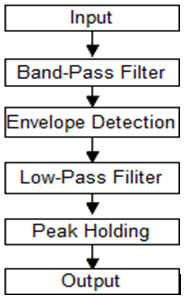Figure 1. The schematic diagram of SPM technology

## 2.png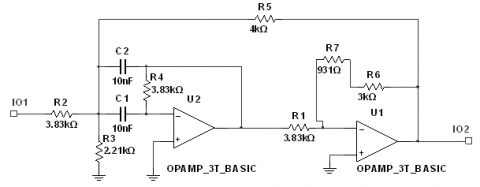Figure 2. The internal structure of the band-passfilter

## 3.pngFigure 3. The internal structure of the envelope detector

## 4.pngFigure 4. The internal structure of the low-pass filter

## 5.png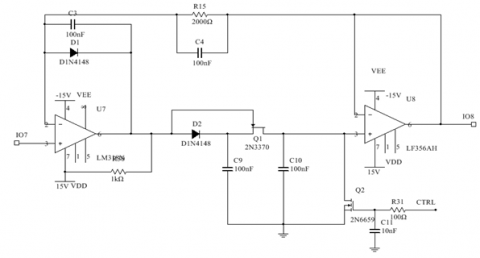Figure 5. The internal structure of the peak holding circuit

The process of extracting the fault signals from the resonance demodulation circuit is divided into three steps:

• High-Q band-pass filter: The broadband fault signal of the input is enhanced by the resonant frequency of installation of the acceleration. Through a high-Q band-pass filter the impact resistance fault signal is reserved while most of conventional interference noise which is distributed in the low frequency band can be eliminated.
• Envelope peak detector: Outer envelope of resonance wave is taken out from envelope detection circuit and the high frequency resonance wave is converted to a low frequency envelope signal.
• Low-pass filter: The high frequency domain interference noise is filtered by the low-pass filter.
• Peak holding circuit: The function of the circuit is to obtain the peak value of the output signal of the voltage and keep the value for a period of time. So that the following circuit has enough time to convert it to digital signal­­ [6-7].
3. The Experimental Results and Analysis

The model of the rolling bearing used in the experiment is N205EM. The bearing characteristic frequency and its calculating formula are shown in Table 1. The significance of each parameter is as follows:

D-Pitch Diameter; d-Roller Diameter; α/°-Contact Angle; z-Roller Number; n-Speed; fs-Sampling Frequency

Table 1. The parameters of bearing

 D/mm d/mm α/° z n/$r\times {{\min }^{-1}}$ fs/Hz 38.5 7.5 0 13 1000 5120

Table 2. The bearing characteristic frequency and its calculating formulas
 Bearing characteristic frequency type Formula for calculating the characteristic frequency of bearing Rotation frequency of inner ring ${{\text{f}}_{r}}=\frac{n}{60}$ Scroll through frequency ${{\text{f}}_{bp}}=\left[ \frac{D}{d}-\left( 1-\frac{d}{D}\cos \alpha \right) \right]{{f}_{r}}$ Inner pass frequency ${{\text{f}}_{ip}}=\frac{Z}{2}\left( 1+\frac{d}{D}\cos \alpha \right){{f}_{r}}$ Outer ring pass frequency ${{\text{f}}_{op}}=\frac{Z}{2}\left( 1-\frac{d}{D}\cos \alpha \right){{f}_{r}}$ Holding rotation frequency ${{\text{f}}_{cp}}=\frac{1}{2}(1-\frac{d}{D}\cos \alpha ){{f}_{r}}$

In the experiment, the sampling frequency is 5120Hz, the center frequency is 1200Hz and the bandwidth is 640Hz. With the increase of the noise amplification, the maximum voltage is changed significantly. The following figures are the inner ring, rolling body and the outer ring of the voltage waveform obtained respectively by SPM circuit magnification to 10, 30, 50. The following values can be obtained by calculating:

## 6.pngFigure 6.  Output waveform for magnification of 10 of inner ring

## 7.png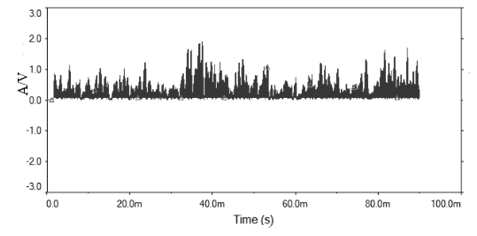Figure 7. Output waveform for magnification of 30 of rolling body

## 8.pngFigure 8. Output waveform for magnification of 10 of inner ring

For different noise amplification cases, three sets of data measured at each magnification of 0,5,10,15... 50. Take the average value and draw out the inner ring, rolling body and the outer ring. The noise magnification - voltage amplitude relation curves are shown in Fig.9-11. It can be obtained from the analysis of the figures:

• With the increase of noise magnification or the decrease of the SNR, the maximum voltage is increased and is similar to the linear relation.
• According to the output voltage amplitude, the fault type can not be determined. The specific fault types need to be combined with other methods to determine because various types of faults are similar.
• Signal to noise ratio is different, the criterion of bearing fault will be different. The signal to noise ratio of the same situation can be in accordance with the same standard.

## 9.png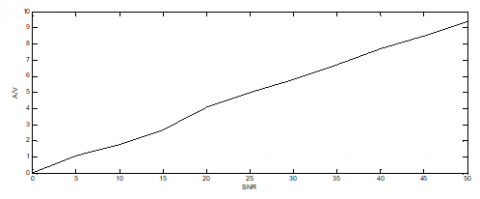Figure 9. The curve of the voltage amplitude- noise amplification factor of inner ring

## 10.pngFigure 10. The curve of the voltage amplitude- noise amplification factor of rolling body

## 11.pngFigure 11. The curve of the voltage amplitude- noise amplification factor of outer ring

4. Criteria for Judging the State of Rolling Bearing

Define the bearing state level by using the dBm that indicates the maximum extent of damage to the rolling element bearing. Use the subtraction of dBm and dBc to analyze the reason of bearing damage. The shock pulse value of bearing is determined as follows ­­ :

• When dBc<10dB, dBm≤20dB, the bearing is in good condition.
• When dBc<dBm≤35dB, the bearing is in deterioration tendency.
• When 35dB<dBm≤50dB, the bearing condition is poor and need to be checked.
• When dBm>50dB, the bearing damage seriously.

## 12.png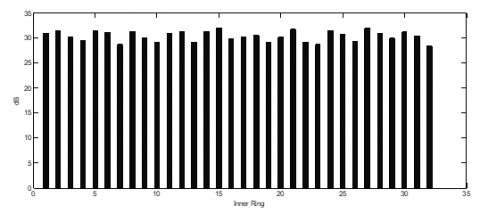Figure 12. The shock pulse value of inner ring

## 13.pngFigure 13. Time domain voltage waveform of inner ring

The output voltage waveform of the fault of the inner ring and its corresponding shock pulse value is shown in Fig.12-13. It can be judged that the bearing is in deterioration tendency. With reference to the definition of dB, it is similar to define dVc for carpet voltage and dVm for maximum voltage. The dVm, maximum value of the shock pulse voltage, is used to define the bearing state level. With n (n≥0) to represent the noise magnification, the judging criterion of using voltage in different noise amplification is concluded as follows:

• When dVc<1V, dVc <dVm≤(1+n/5)V, the bearing is in good condition.
• When dVc<1V, (1+n/5)V<dVm≤(2+n/5)V, the bearing is in deterioration tendency.
• When dVc<1V, (2+n/5)V<dVm≤(3+n/5), the bearing condition is poor and need to be checked.
• When dVc<1V, dVm>(3+n/5)V, the bearing damages seriously.
5. Conclusions

In this paper, the traditional shock pulse signal processing is improved. The pulse sequence generation circuit made up by the peak holding circuit after the early signal processing(band-pass filtering, envelope detection, low-pass filtering) to generate the rolling bearing shock pulse signal. The adjustment laws of dVm value of the output under different noise amplification conditions is also discussed. Through the above analysis, the following conclusions can be drawn:

• The influence of the noise amplification factor on the SPM circuit is remarkable. With the increase of the noise amplification or the SNR reduced, the amplitude of dVm is increased and is similar to linear relation.
• The influence of noise must be taken into account if the shock pulse voltage maximum value of dVm is used to judge bearing state.

The state of the bearing can be judged roughly based on the SPM circuit. The specific fault types can be diagnosed in more detail with the help of other auxiliary methods.

Acknowledgment

This research is supported by National Natural Science Foundation of China (11227201, 11372199, 11572206) and Natural Science Foundation of Hebei Province (A2014210142).

References

 Mei Hongbin, Vibration monitoring and diagnosis of rolling bearing -- Theory, Method &System. Beijing: Machinery Industry Press,1996.

 Wang Xiaoping and Chen Yaowu, SPM method for fault diagnosis of rolling bearing, Mechanical & Electrical Engineering Magazine, vol. 3, pp. 3l-32, 1996.

 Yi Liangju, Simple Vibration Diagnosis Technology, Beijing: Mechanical Industry Press, 2003

 Zhao Wei, “Fault diagnosis of rolling bearing by shock pulse method,” Bearing, vol. 5, pp. 31-33, 2006.

 Zhang Ying, Lv Luyong and Wan Shuting, “The shock pulse method applied on fault diagnosis of rolling bearing,” Petroleum-Chemical Equipment Technology, vol. 28, no. 4, pp. 60-64, 2007.

 Huang Baoming and Huanghai, “The improvement and application of real-time on-line rolling bearing monitoring instrument based on shock pulse method,” Journal of Electronic Measurement and Instrument, vol. 15, no. 2, pp. 22-25, 2001.

 Li Shanshan, Design and Improvement of Portable Bearing Fault Detector Based on Shock Pulse Method, Instrument  Technique and Sensor, 2014 (4): 106-107.

 Shen Qinggen, Equipment Fault Diagnosis, Beijing: Chemical Industry Press, 2006.